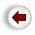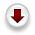# 2. B-trees: Perfectly Height-balanced M-way search trees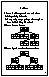A B-tree is an M-way search tree with two special properties:

1. It is perfectly balanced: every leaf node is at the same depth.

2. Every node, except perhaps the root, is at least half-full, i.e. contains M/2 or more values (of course, it cannot contain more than M-1 values). The root may have any number of values (1 to M-1).
The 3-way search tree above is clearly not a B-tree. Here is a 3-way B-tree containing the same values:
`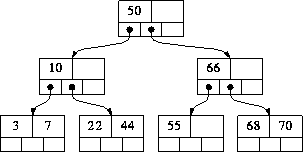`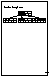And here is a 5-way B-tree (each node other than the root must contain between 2 and 4 values):

`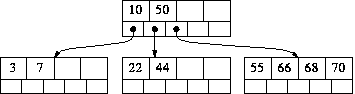`

In the descriptions of our algorithms, we assume that M is odd; therefore each node (other than the root) must contains between (M-1)/2 and M-1 values.